# Isosceles Triangle - Definition with Examples

The Complete K-5 Math Learning Program Built for Your Child

• 40 Million Kids

Loved by kids and parent worldwide

• 50,000 Schools

Trusted by teachers across schools

• Comprehensive Curriculum

Aligned to Common Core

What is Isosceles Triangle?

A triangle with two sides of equal length is an isosceles triangle. The two equal sides of an isosceles triangle are known as ‘legs’ whereas the third or unequal side is known as the ‘base’.

Angles opposite to equal sides in an isosceles triangle are always of equal measure. In the given isosceles triangle, if AB = AC then ∠B = ∠C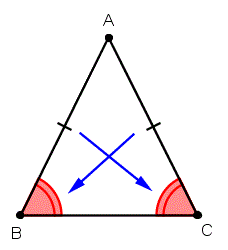Here are a few examples of the isosceles triangle: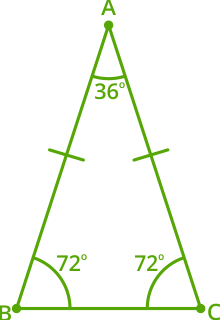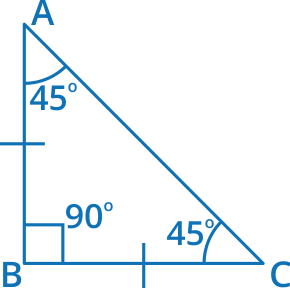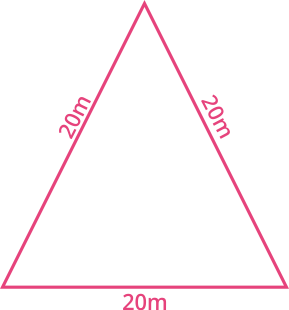Real life examples

Many things in the world have the shape of an isosceles triangle.  Some popular examples of an isosceles triangle in real life are a slice of pizza, a pair of earrings.Non-examples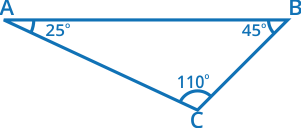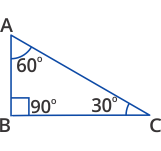General properties

• The equal sides of an isosceles triangle are known as the ‘legs.’

• The third and unequal side of an isosceles triangle is known as the ‘base.’

• The angle made by the two equal sides of an isosceles triangle is known as the ‘vertex angle.’

• The angles that involve the base of an isosceles triangle are known as the ‘base angles.’

• The angles situated opposite to the equal sides of an isosceles triangle are always equal.

• All the three angles situated within the isosceles triangle are acute, which signifies that the angles are less than 90°.

• The sum of three angles of an isosceles triangle is always 180°, which means we can find out the third angle of a triangle if the two angles of an isosceles triangle are known.

 Fun Facts The term isosceles triangle is derived from the Latin word ‘īsoscelēs’, and the ancient Greek word ‘ἰσοσκελής (isoskelḗs)’ which means “equal-legged”. Moreover, ancient Babylonian and Egyptian mathematics knew how to calculate ‘area’ long before the ancient Greek mathematicians studied the isosceles triangle. The isosceles shape of the buildings not only makes them attractive but also earthquake-resistant.

Won Numerous Awards & Honors